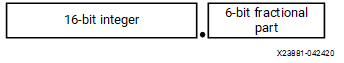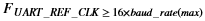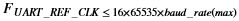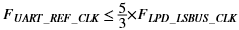# Baud Rate Divider

## Versal ACAP Technical Reference Manual (AM011)

Document ID
AM011
Release Date
2022-12-16
Revision
1.5 English

The baud rate divisor is a 22-bit number consisting of a 16-bit integer and a 6-bit fractional part. This is used by the baud rate generator to determine the bit period. The fractional baud rate divider enables the use of any clock with a frequency >3.6864 MHz to act as UARTx_REF_CLK, while it is still possible to generate all the standard baud rates.

The 16-bit integer is written to the Integer Baud Rate bit field, UARTx.BAUD_INTEGER [DIVINT]. The 6-bit fractional part is written to the Fractional Baud Rate bit field, UARTx.BAUD_FRACT [DIVFRAC]. The baud rate divisor has the following relationship to UARTx_REF_CLK:

Baud Rate Divisor = UARTx_REF_CLK/(16× Baud Rate) = [DIVINT] . [DIVFRAC]

Figure 1. Baud Rate DivisorThe 6-bit number (m) can be calculated by taking the fractional part of the required baud rate divisor and multiplying it by 64 (that is, 2^[DIVFRAC]) and adding 0.5 to account for rounding errors:

m = integer ([BAUD_FRACT] × 2^n + 0.5)

An internal clock enable signal, Baud16, is generated, and is a stream of one UARTx_REF_CLK wide pulses with an average frequency of 16 times the required baud rate. This signal is then divided by 16 to give the transmit clock. A low number in the baud rate divisor gives a short bit period, and a high number in the baud rate divisor gives a long bit period.

## Baud Rate Clock

The frequency selected for UARTx_REF_CLK must accommodate the required range of baud rates:

••For example, for a range of baud rates from 110 baud to 460800 baud the UARTx_REF_CLK frequency must be between 7.3728 MHz to 115.34 MHz. The frequency of UARTx_REF_CLK must also be within the required error limits for all baud rates to be used. There is also a constraint on the ratio of clock frequencies for LPD_LSBUS_CLK to UARTx_REF_CLK. The frequency of UARTx_REF_CLK must be no more than 5/3 times faster than the frequency of LPD_LSBUS_CLK:

•For example, in UART mode, to generate 921600 baud when UARTx_REF_CLK is 14.7456 MHz, LPD_LSBUS_CLK must be greater than or equal to 8.85276 MHz. This ensures that the UART has sufficient time to write the received data to the receive FIFO.